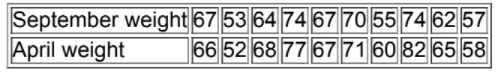×
Get Full Access to Elementary Statistics - 12 Edition - Chapter 13.3 - Problem 1 bsc
Get Full Access to Elementary Statistics - 12 Edition - Chapter 13.3 - Problem 1 bsc

×

# Solved: Wilcoxon Signed-Ranks Test for Freshman 15TheISBN: 9780321836960 18

## Solution for problem 1 BSC Chapter 13.3

Elementary Statistics | 12th Edition

• Textbook Solutions
• 2901 Step-by-step solutions solved by professors and subject experts
• Get 24/7 help from StudySoup virtual teaching assistantsElementary Statistics | 12th Edition

4 5 1 322 Reviews
17
4
Problem 1 BSC

Wilcoxon Signed­Ranks Test for Freshman 15 The following table lists some of the weights from Data Set 4 in Appendix B. Those weights were measured from college students in September and later in April of their freshman year. Assume that we plan to use the Wilcoxon signed­-ranks test to test the claim of no difference between September weights and April weights. What requirements must be satisfied for this test? Is there any requirement that the populations must have a normal distribution or any other specific distribution? In what sense is this Wilcoxon signed­-ranks test a “distribution­-free test”?Step-by-Step Solution:
Step 1 of 3

Solution 1BSC

In order to use the Wilcoxon sign-ranks test the following requirements must be satisfied:

(i) The data are a simple random sample.

(ii) The population of differences has a distribution that is approximately symmetric.

While using the Wilcoxon sign-ranks test, the sign of the differences and the magnitude of the difference is taken in to the account. So there is no requirement that the sample data came from a population with any particular distribution, such as normal distribution.

Wilcoxon sign-ranks test is a distribution free test; it’s used for the data which does not follow the normal distribution. Here only the sign of the difference and the magnitude of the signs is checked.

Step 2 of 3

Step 3 of 3

##### ISBN: 9780321836960

This full solution covers the following key subjects: test, weights, ranks, wilcoxon, Signed. This expansive textbook survival guide covers 121 chapters, and 3629 solutions. The full step-by-step solution to problem: 1 BSC from chapter: 13.3 was answered by , our top Statistics solution expert on 03/15/17, 10:30PM. The answer to “?Wilcoxon Signed­Ranks Test for Freshman 15 The following table lists some of the weights from Data Set 4 in Appendix B. Those weights were measured from college students in September and later in April of their freshman year. Assume that we plan to use the Wilcoxon signed­-ranks test to test the claim of no difference between September weights and April weights. What requirements must be satisfied for this test? Is there any requirement that the populations must have a normal distribution or any other specific distribution? In what sense is this Wilcoxon signed­-ranks test a “distribution­-free test”?” is broken down into a number of easy to follow steps, and 97 words. Elementary Statistics was written by and is associated to the ISBN: 9780321836960. Since the solution to 1 BSC from 13.3 chapter was answered, more than 345 students have viewed the full step-by-step answer. This textbook survival guide was created for the textbook: Elementary Statistics, edition: 12.

## Discover and learn what students are asking

Statistics: Informed Decisions Using Data : Data Collection
?In Problems 1–5, provide a definition using your own words. 1. Statistics

Statistics: Informed Decisions Using Data : The Randomized Complete Block Design
?Given the following ANOVA output, answer the questions that follow: (a) The researcher wants to test H0: m1 = m2 = m3 =

Unlock Textbook Solution